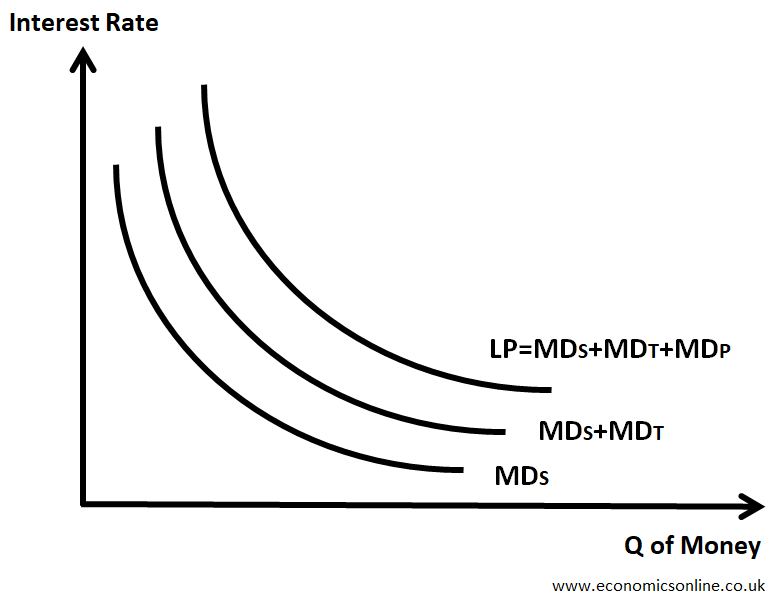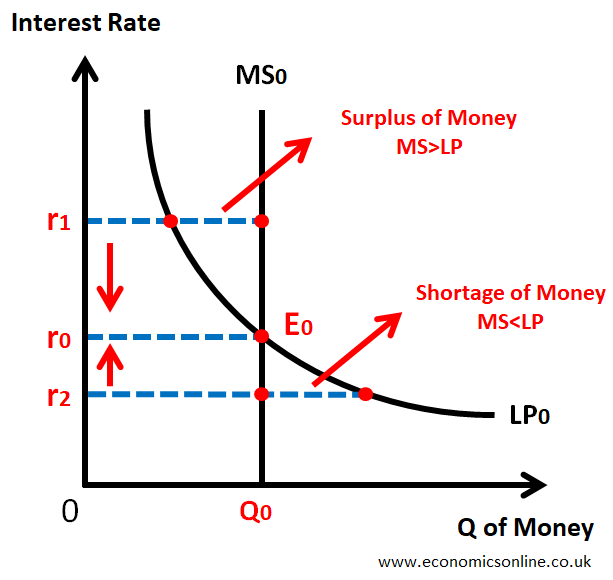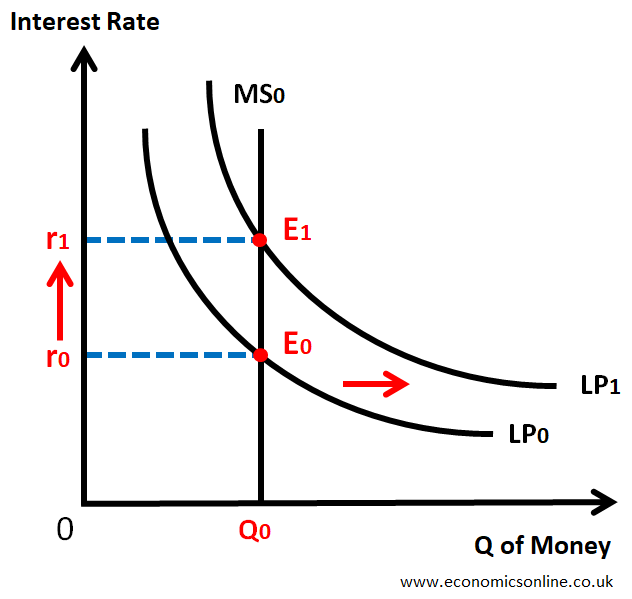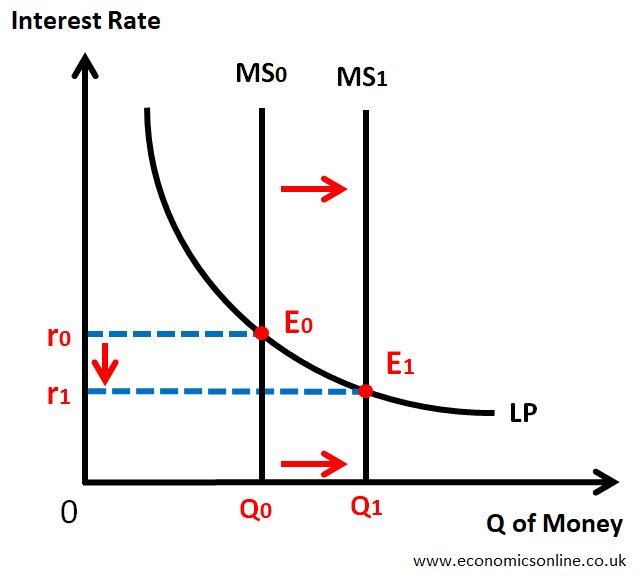# Liquidity Preference Theory with Graphs

## Introduction

The interest rate is the cost of borrowing money, expressed as a percentage of the loan amount which is charged by the lenders from the borrowers. In financial markets, the determination of interest rate is an important concept to understand the workings of the money market and the behaviour of economic agents in that market. There are many theories of interest rate determination. One is the loanable funds theory of interest rate determination, which is already explained in another article. In this article, we will look at the liquidity preference theory in detail.

## What is Liquidity Preference Theory?

Liquidity preference theory refers to the determination of the interest rate by using the demand for money and the supply of money in the money market of a country.

It is also called Keynesian liquidity preference theory and is considered as an important topic in modern economics.

## Origin

This theory was proposed by the economist John Maynard Keynes in 1936. The book in which Keynes mentioned the liquidity preference theory is “The General Theory of Employment, Interest, and Money,” which was published in 1936. According to J. M. Keynes, the interest rate, just like the price of any commodity, is determined by the forces of demand for and supply of money in the money market.

## What is Liquidity?

Liquidity refers to the ease or speed with which an asset can be converted into cash. Cash is the most liquid asset. Bank deposits are also considered highly liquid.

## Assumptions of Liquidity Preference Theory

Liquidity preference theory has the following assumptions:

• People and firms hold all their wealth in two ways, i.e., cash and bonds.
• Financial institutions (banking system) are well established.
• The supply of money is fixed.
• The same interest rate is charged for all types of financial assets.
• Demand for money for transactionary and precautionary motives is perfectly interest-inelastic.
• Everyone speculates.

## The Interest Rate Determination

According to the liquidity preference theory, the interest rate is determined by

1. Demand for money (MD) of Liquidity Preference (LP)
2. Supply of Money (MS)

## Demand for Money or Liquidity Preference

The willingness of people and firms to hold cash (prefer liquidity) is called liquidity preference (LP). This is also called demand for money (MD).

Demand for money or liquidity preference is due to three motives.

1. Transactionary Motive
2. Precautionary Motive
3. Speculative Motive

### Demand for Money for Transactionary Motive (MDT)

People hold cash (prefer liquidity) for transactionary motives to do daily transactions for a certain period of time. Cash is needed for day-to-day transactions, as money is the medium of exchange.

• Households need cash to buy food, clothing, to pay bills.
• Firms need cash to buy raw materials, pay wages, etc.
• The government needs cash to meet the daily expenditures of government offices.

Money demand for transactional motive (MDT) is perfectly interest-inelastic because households, firms, and governments have to fulfil their day-to-day transactions irrespective of the value of the prevailing interest rate.

The following graph shows this.

The vertical MDT curve shows that if the interest rate is increased from r1 to r2, the quantity of money that people hold will remain the same at Q0, making this curve perfectly interest-inelastic.

Determinants of Demand for Money for Transactionary Motive

The following factors affect the transaction demand for money.

1. Level of Income

An increase in income means people will hold more money for transactionary motive and vice versa.

2. Inflation

If the general price level goes up, things will generally be expensive, and more money will be needed to buy the same quantity of goods and services.

3. Income Intervals

If people receive income after long intervals (say months instead of weeks), they need to hold more cash for the transaction of the whole month, and hence the demand for money for transactionary motive will be high.

4. Uncertainty

In case of higher uncertainty (for example, economic instability), people prefer to hold more cash, leading to a higher demand for money for transactionary motive.

### Demand for Money for PrecautionaryMotive (MDP)

People hold cash (prefer liquidity) for future uncertainties (precautionary motive), such as to tackle emergencies and sudden losses.

• Households keep cash for job loss, theft, accidents, unexpected family expenses, illness, etc.
• Firms keep cash for fire, theft, accidents, machinery breakdowns, losses, etc.

Money demand for precautionary motive (MDP) is perfectly interest-inelastic because households and firms have to keep some cash for emergencies irrespective of the value of the prevailing interest rate.

The following graph shows this.

The vertical MDP curve shows that if the interest rate is increased from r1 to r2, the quantity of money that people hold will remain the same at Q0, making this curve perfectly interest-inelastic.

Determinants of Demand for Money for Precautionary Motive

The following factors affect the precautionary demand for money.

1. Level of Income

An increase in income means people will hold more money for precautionary motive and vice versa.

2. Inflation

If the general price level goes up, things will generally be expensive, and more money will be needed to fund any upcoming emergency.

3. Income Intervals

If people receive income after long intervals (say months instead of weeks), they need to hold more cash as an emergency fund for the whole month, and hence the demand for money for precautionary motive will be high.

4. Uncertainty

In case of higher uncertainty (for example, economic instability), people prefer to hold more cash for emergencies, leading to a higher demand for money for precautionary motive.

### Demand for Money for SpeculativeMotive (MDS)

Speculation means the purchase of an asset for short-term gain due to the difference between its sale price and purchase price. Liquidity preference theory assumes that people will hold only two forms of assets, i.e. cash and bonds.

Bonds are certificates issued by the government at a discounted rate (i.e., less than their face value) for a long period (usually more than one year), and they have a fixed amount of interest per annum regardless of their market price.

People expect to have certain future rewards by investing money in bonds, so they hold cash for that purpose leading to the speculative demand for money.

The following formula gives the inverse relationship between the market price of bonds and the interest rate.A image of a formula showing the relationship between interest rate the the price of bonds.

Let us understand this with the following table.

 Initial Final Price of Bond \$100 \$200 Fixed Amount of Interest \$10 \$10 Interest Rate 10% 5%

This table shows that the fixed amount of interest of \$10 is paid on bonds. If the price of bonds in the bond market goes up from \$100 to \$200, the market rate of interest will go down from 10% [(\$10/\$100) x 100%] to 5% [(\$10/\$200) x 100%].

This negative relationship between the bond prices and interest rate gives rise to the following downward sloping and interest elastic curve of demand for money for speculative motive.

In the above graph, at a higher interest rate (r1), the price of bonds will be low, and there will be high opportunity cost of holding cash. So, people will buy bonds, and they will hold less cash. So, MDS will be low (Q1).

When the interest rate is low (r2), the price of bonds will be high, and there will be low opportunity cost of holding cash. So, people will sell bonds, and they will hold more cash. So, MDS will be high (Q2).

### Total Demand for Money or Liquidity Preference (LP)

Total Demand for Money or Liquidity Preference (LP) is the sum of the following three components.

1. Demand for money for transactionary motive (MDT)
2. Demand for money for precautionary motive (MDP)
3. Demand for money for speculative motive (MDS)

LP = MDT + MDP + MDS

The following graph illustrates this.A graph illustrating the total demand for money or liquidity preference.

In the above graph, the LP curve shows the total demand curve for money, and we will use this LP curve for interest rate determination.

## Supply of Money (MS)

The supply of money refers to the total amount of money available in an economy.

In liquidity preference theory, the supply of money (MS) is assumed to be fixed in the short run because it is controlled by the central bank of the country. The following graph shows this.

## The Equilibrium Interest Rate

It is an interest rate for which the demand for money and the supply of money are equal, and there is no tendency to change. That is, LP = MS.

In other words, the interaction between the demand for and supply of money determines the equilibrium interest rate, which is the rate at which the demand for money equals the supply of money.

Let us illustrate this with the following diagram.A graph illustrating the interest rate determination in liquidity preference theory.

In the above graph, the quantity of money is taken on the horizontal axis (x-axis), and interest rate is taken on the vertical axis (y-axis).

The market equilibrium is at E0, which is the point of intersection of the demand for money or liquidity preference (LP0) and the supply of money (MS0). r0 is the equilibrium interest rate

At r1, MS>LP, which means the surplus of cash. So people will buy bonds to utilise that cash due to high opportunity cost of holding cash. So, the buying of bonds will increase, leading to a rise in the demand for bonds in the bond market. This will increase the price of bonds, and the interest rate will decrease from r1 to r0 due to its inverse relationship with the price of bonds. Hence, market equilibrium will be restored at r0.

At r2, MS<LP, which means the shortage of cash. So, people will sell bonds to have more cash. So selling of bonds will increase, leading to a rise in the supply of bonds in the bond market. This will decrease the price of bonds, and the interest rate will increase from r2 to r0 due to its inverse relationship with the price of bonds. Hence, market equilibrium will be restored at r0.

## Changes in Equilibrium Interest Rate

The equilibrium interest rate can be changed due to a shift in the demand for money, a shift in the supply of money or the simultaneous shift of both the demand for and the supply of money. Let us discuss a couple of cases.

### Rise in the Demand for Money or Liquidity Preference (LP)A graph illustrating the change in interest rate in liquidity preference theory due to rise in the demand for money.

In the above graph, the liquidity preference curve is shifted towards the right from LP0 to LP1, leading to a rise in interest rate from r0 to r1.

### Rise in the Supply of MoneyA graph illustrating the change in interest rate in liquidity preference theory due to rise in the supply of money.

In the above graph, the money supply is shifted towards the right from MS0 to MS1, leading to a fall in interest rate from r0 to r1.

## Liquidity Trap

Liquidity Trap is a situation when an increase in money supply (MS) has no effect on the interest rate.

Normally, an increase in the money supply will cause interest rate to fall. However, at a low interest rate, liquidity preference (LP) becomes perfectly interest elastic, and an increase in money supply (MS) does not affect the interest rate. Keynes described this situation as a liquidity trap.A graph illustrating the liquidity trap according to the liquidity preference theory.

In the above graph, the liquidity is shown from Q0 to Q2, where the increase in the money supply from MS0 to MS1 and MS2 does not affect the interest rate, and it remains the same at r0.

## Limitations of Liquidity Preference Theory

The liquidity preference theory has the following limitations.

• People and firms do not hold all their wealth in two ways, i.e. cash and bonds.
• The financial system in less developed countries (LDCs) is not well established.
• The same interest rate is not charged for all types of financial assets.
• Money demand for transactionary and precautionary motives is not perfectly interest inelastic, especially at high interest rates.
• Everyone does not speculate.

## Criticism

The criticisms of the liquidity preference theory are:

### Simplistic View of Interest Rate Determination

Some critics say that the liquidity preference theory simplifies the view of interest rate determination by only focusing on the demand for and supply of money. They argue that loanable funds (as per the loanable funds theories of interest) and other investment opportunities also impact interest rate determination.

### Neglect of Investment and Productivity

This theory neglects the relationship between the interest rate and investment and productivity. Critics argue that there is not only the demand for money that impacts interest rates but also investment decisions and economic productivity, which are a country's key factors of economic activity and growth.

### Lack of Empirical Evidence

Critics argue that there is no empirical evidence to support liquidity preference theory. The relationship between interest rates and demand for money is complex and influenced by other factors.

### Incomplete Treatment of the Role of Expectations

Critics argue that the liquidity preference theory does not provide a complete framework to understand the economic behaviour and role of expectations. It provides an incomplete treatment of the role of expectations.

## Conclusion

In conclusion, Keynes has provided us with a useful framework for understanding the process of interest rate determination in the money market in the form of the liquidity preference theory of interest. The liquidity preference theory tells us that the interest rate is determined in the money market in the same way as the price of any commodity, that is, by using the demand and supply forces. However, others criticised it because it oversimplifies the interest rate, neglects investments and productivity, and provides incomplete treatment of the role of expectations. However, liquidity preference theory helps us understand the preference of individuals and businesses to hold money instead of other assets.